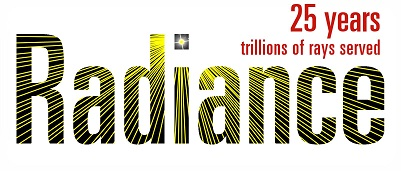# Point Cloud Reuse

Hi

I have pre-rendered data:

X/Y/Z intersection point for each ray, normal for each intersection and reflectivity (assumed Lambertian surfaces). I’d like to add a light source and see how the result would change without having to do a full render. Can Radiance somehow reuse this data? I realize that only direct lighting is possible since we can’t calculate new intersection points on surfaces.

Thanks!

Yes, that’s certainly possible, though as I think you observed, you won’t get correct shadows from such a post-process. For example, you could create a “deep pixel” image with rtrace like so:

``````vwrays -ff -x 1024 -y 1024 [view] | rtrace -fff -ovpn [options] octree > deepimg.flt
``````

Then process it with rcalc:

``````rcalc -if9 -of -e 'ri=\$1;gi=\$2;bi=\$3;px=\$4;py=\$5;pz=\$6;nx=\$7;ny=\$8;nz=\$9' -f clight.cal -e '\$1=ri+rl;\$2=gi+gl;\$3=bi+bl' deepimg.flt | pvalue -h -r -df -Y 1024 +X 1024 > lightadded.hdr
``````

Your “clight.cal” file would define how the variables “rl”, “gl” and “bl” are computed from the intersection location (px,py,pz) and surface normal (nx,ny,nz). Normally, this would simply be the direction vector between the source and intersection point, and a dot product with the surface normal, times the light source radiance in red, green, and blue, respectively, times the source area divided by the square of the distance. For example:

``````{ Source position }
spx1 : 5; spy1 : 7; spz1 : 4;
{ Source area }
area1 : 0.58;
L1r : 1000; L1g : 900; L1b : 800;
{ Calculate contribution from light 1 }
vx1 = spx1 - px;
vy1 = spy1 - py;
vz1 = spz1 - pz;
d1sq = vx1*vx1 + vy1*vy1 + vz1*vz1;
dotp = (vx1*nx + vy1*ny + vz1*nz)/sqrt(d1sq);
m1 = if(dotp, dotp*area1/d1sq, 0);
rl = L1r*m1;
gl = L1g*m1;
bl = L1b*m1;
``````

Keep in mind that light area is in squared world units, and should correspond to the parallel projection of a source that is assumed to be round(ish). So, a spherical source of radius 5 would have a projected area of 25pi, for example.

The rtrace command is meant to be illustrative only – you would actually use the surface color in place of the computed pixel color in your case, or modify the calculation to take the diffuse reflectivity into account some other way.

-Greg

Coming back to this topic after a whileI wonder if there’s a shortcut I could take here.
For example, what if I use the -I option in `rtrace`, and provide the intersections and normals as sensor locations/normals?

In a first attempt that seems like it might work (the image is different by a constant factor from what I get in a normal render). Is there some pitfall I may encounter by doing that?

Certainly. The -I+ calculation in rtrace yields irradiance values, which equals radiance from a diffuse white surface that somehow had the impossible reflectance of 3.1416 (pi). Therefore, you can multiply this output by actual_reflectance/pi to get the correct radiance from a surface with that orientation and specified actual_reflectance. (The calculation is repeated individually per color channel.)